Next: About this document ... Up: lab_template Previous: lab_template

Subsections

# Taylor Polynomials

## Purpose

The purpose of this lab is to use Maple to introduce you to Taylor polynomial approximations to functions, including some applications.

## Getting Started

To assist you, there is a worksheet associated with this lab that contains examples similar to some of the exercises. On your Maple screen go to File - Open then type the following in the white rectangle:
\\storage\academics\math\calclab\MA1023\Taylor_powerseries_start_B16.mw

You can copy the worksheet now, but you should read through the lab before you load it into Maple. Once you have read through the exercises, start up Maple, load the worksheet, and go through it carefully. Then you can start working on the exercises.

## Background

The idea of the Taylor polynomial approximation of order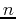at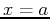, written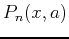, to a smooth functionis to require thatandhave the same value at. Furthermore, their derivatives atmust match up to order. For example the Taylor polynomial of order three for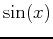at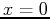would have to satisfy the conditions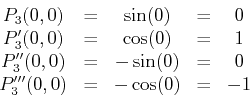You should check for yourself that the cubic polynomial satisfying these four conditions is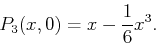The general form of the Taylor polynomial approximation of ordertois given by the following

Theorem 1   Suppose thatis a smooth function in some open interval containing. Then theth degree Taylor polynomial of the functionat the pointis given by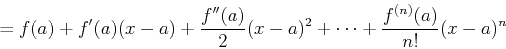We will be seeing this formula a lot, so it would be good for you to memorize it now! The notationis used in the definition to stand for the value of the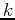-th derivative of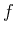at. That is,,, and so on. By convention,. Note that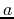is fixed and so the derivativesare just numbers. That is, a Taylor polynomial has the form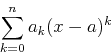which you should recognize as a power series that has been truncated.

## The Ratio Test

The Ratio Test for convergence of a series can be thought of as a measurement of how fast the series is increasing or decreasing. This can be found by looking at the ratio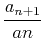as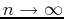. Given the series, suppose that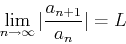Given the series, suppose thatThen

1. the series converges if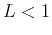,
2. the series diverges if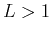,
3. the test is inconclusive if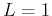.

## Radius or Interval of Convergence

The radius of convergence of a series can usually be found by using the ratio test: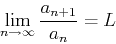Next, you would need to solve for the interval of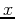values such that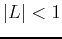.

## Accuracy and Tolerance

To measure how well a Taylor Polynomial approximates the function over a specified interval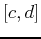, we define the toleranceofto be the maximum of the absolute errorover the interval.

## Maple Commands

To use the Taylor and TayPlot commands you need to load the CalcP7 package.
>with(CalcP7);


The exponential function can be approximated at a base point zero with a polynomial of order four using the following command.

>Taylor(exp(x),x=0,4);

You might want to experiment with changing the order. To see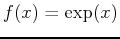and its fourth order polynomial use
>TayPlot(exp(x),x=0,{4},x=-4..4);

This plots the exponantial and three approximating polynomials.
>TayPlot(exp(x),x=0,{2,3,4},x=-2..2);

Notice that the further away from the base point, the further the polynomial diverges from the function. the amount the polynomial diverges i.e. its error, is simply the difference of the function and the polynomial.
>plot(abs(exp(x)-Taylor(exp(x),x=0,3)),x=-2..2);

This plot shows that in the domain x from -2 to 2 the error around the base point is zero and the error is its greatest at x = 2 with a difference of over one. You can experiment with the polynomial orders to change the accuracy. If your work requires an error of no more than 0.2 within a given distance of the base point then you can plot your accuracy line y = 0.2 along with the difference of the function and the Taylor approximation polynomial.
>plot([0.2,abs(exp(x)-Taylor(exp(x),x=0,3))],x=-2..2,y=0..0.25);

We knew this would have some of its error well above 0.2. Change the order from three to four. As you can see there are still some values in the domain close to x = 2 whose error is above 0.2. Now try an order of 5. Is the error entirely under 0.2 between x = -2 and x = 2? Larger orders will work as well but order five is the minimum order that will keep the error under 0.2 within the given domain.

## Exercises

1. Use Maple to find the interval of convergence for the following series
a)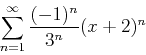b)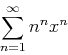2. Find the power series for each function listed below aboutand then determine the interval ofvalues over which the series converges.

1.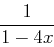2.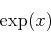3.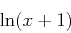3. For the function,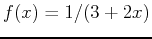, use the TayPlot command to plot the function and multiple Taylor polynomial approximations of various orders with base point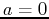on the same graph over the interval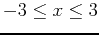; use a y-range from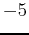to.
a)
If you increase the order of the Taylor polynomial, can you get a good approximation at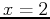?
b)
Estimate the interval of convergence based on the plot and compare to the actual interval of convergence as done in the previous exercise.

4. For the following functions and base points, determine what minimum order is required so that the Taylor polynomial approximates the function to within a tolerance of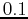over the given interval.
a), base point, interval.
b)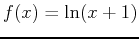, base point, interval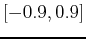.
c)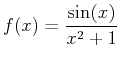, base point, interval.Next: About this document ... Up: lab_template Previous: lab_template
Dina J. Solitro-Rassias
2016-12-01